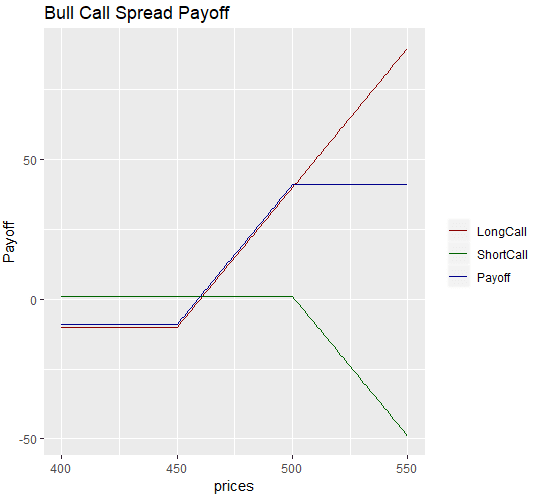# Options Strategy: Create Bull Call Spread with R Language

Bull Call Spread is an options trading strategy that involves the purchase of two call options with the same expiration and different strike prices. In the strategy, the trader buys one call option with a lower strike price and sells another call option with a higher strike price. Both calls have the same underlying security. The strategy has a limited potential profit and loss as it has a ceiling for the profits and a floor for losses. In this article we will simulate the bull call spread with R.

The inputs and the strategy code for bull call spread with R are provided below. Note that for the purpose of the example, we are generating our own data, i.e., a sequence of numbers using the seq() function in R. We create the strategy with two call options one with a low strike price of 450 and another with a high strike price of 500. We also have the premium amounts of these two. We calculate the intrinsic value and payoff from each call option and then we calculate the strategy payoff. Then we plot the strategy payoff at different prices using the ggplot function in R.

prices <- seq(400, 550,1) # Vector of prices
k_low = 450  # Low Strike price for call
k_high = 500 # High Strike Price for call
# Intrinsic Value and Payoff long call
intrinsicValueLongCall <- prices - k_low - premium_low
# Intrinsic Value and Payoff short call
intrinsicValueShortCall <- prices - k_high - premium_high
# Strategy Payoff
payoff <- rowSums(cbind(payoffLongCall,payoffShortCall))f
# Generate a dataframe with the payoffLongCall, payoffShortCall and payoff vectors
# in order to plot the strategy payoffs using ggplot
results <- data.frame(cbind(payoffLongCall,payoffShortCall,payoff))
ggplot(results, aes(x=prices)) +
geom_line(aes(y = payoffLongCall, color = "LongCall")) +
geom_line(aes(y = payoffShortCall, color="ShortCall"))+
geom_line(aes(y=payoff, color = 'Payoff')) +
scale_colour_manual("",
breaks = c("LongCall", "ShortCall", "Payoff"),
values = c("darkred", "darkblue", "darkgreen")) + ylab("Payoff")+